Express each of the following quadratic functions in the form of f(x) = a (x – h)²+ k.Then,state the minimum or maximum value,axis of symmet

Question

Express each of the following quadratic functions in the form of f(x) = a (x – h)²+ k.Then,state the minimum or maximum value,axis of symmetry and minimum or maximum point. (a) f(x) = -2x² + 7x + 4.
Pls help me! I’ll mark u as the brainliest​

in progress 0
5 months 2021-08-04T12:11:19+00:00 1 Answers 6 views 0

1. Given:

The function is: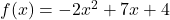To find:

Express the quadratic equation in the form of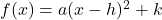, then state the minimum or maximum value,axis of symmetry and minimum or maximum point.

Solution:

The vertex form of a quadratic function is:…(i)

Where, a is a constant, (h,k) is the vertex and x=h is the axis of symmetry.

We have,It can be written as: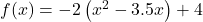Adding and subtracting square of half of coefficient of x inside the parenthesis, we get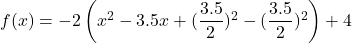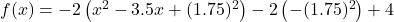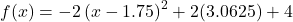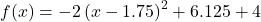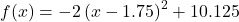…(ii)

On comparing (i) and (ii), we get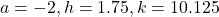Here, a is negative, the given function represents a downward parabola and its vertex is the point of maxima.

Maximum value = 10.125

Axis of symmetry :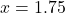Maximum point = (1.75,10.125)

Therefore, the vertex form of the given function is, the maximum value is 10.125, the axis of symmetry isand the maximum point is (1.75,10.125).